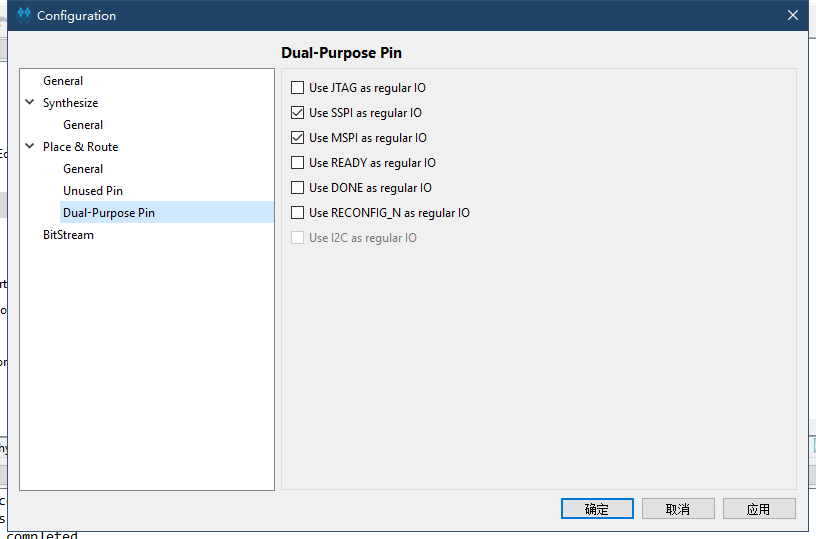# RGB LCD 示例

## 先介绍时序

RGB LCD 显示协议和 VGA 类似，通信都有专用的行同步、场同步信号线。它们的主要区别是前者传输用的是数字信号，后者传输走的是模拟信号。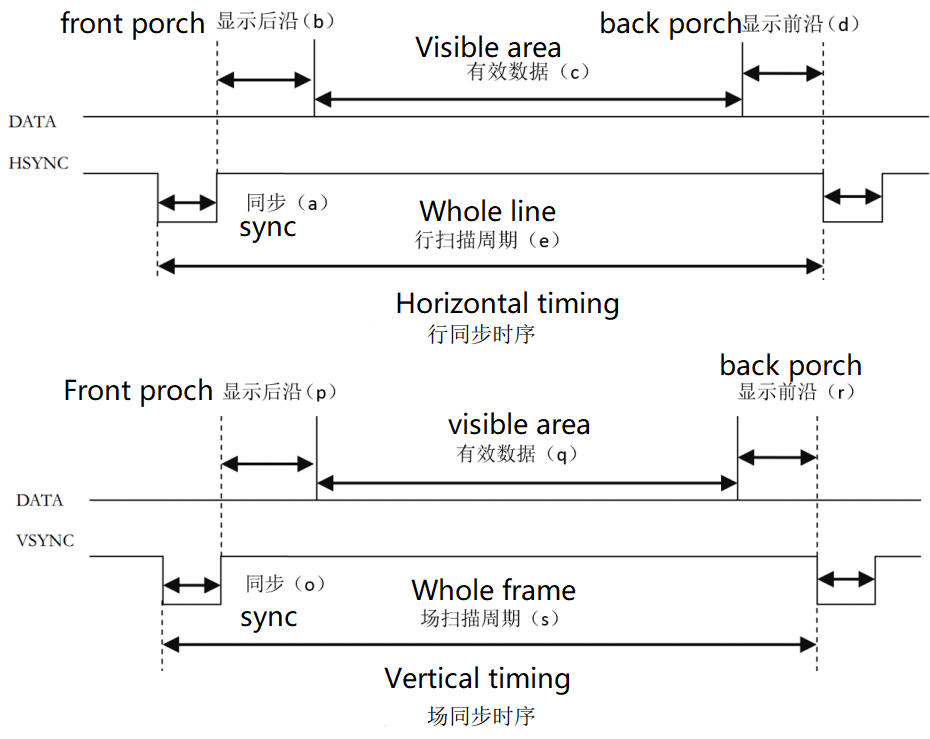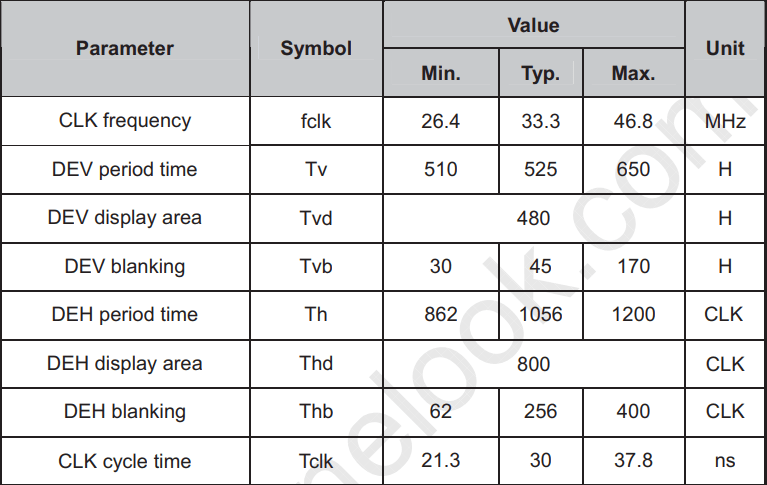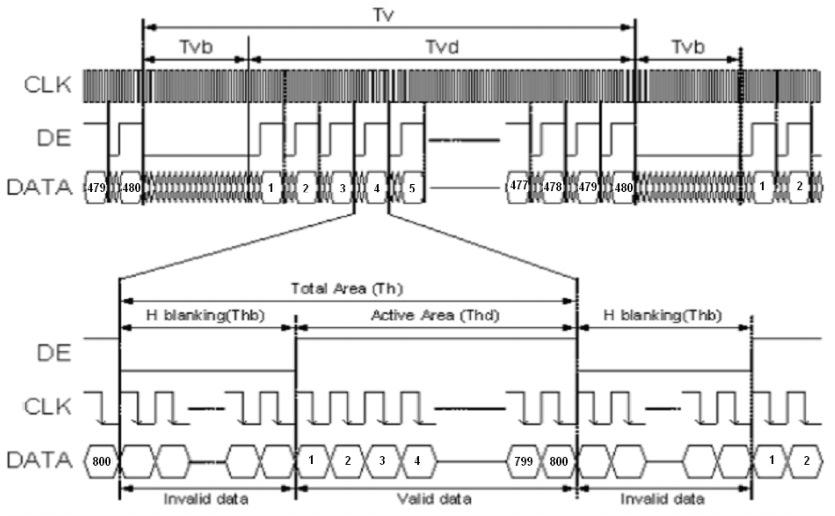## 生成屏幕时钟

• 这里需要用到高云官方的IP核

### pll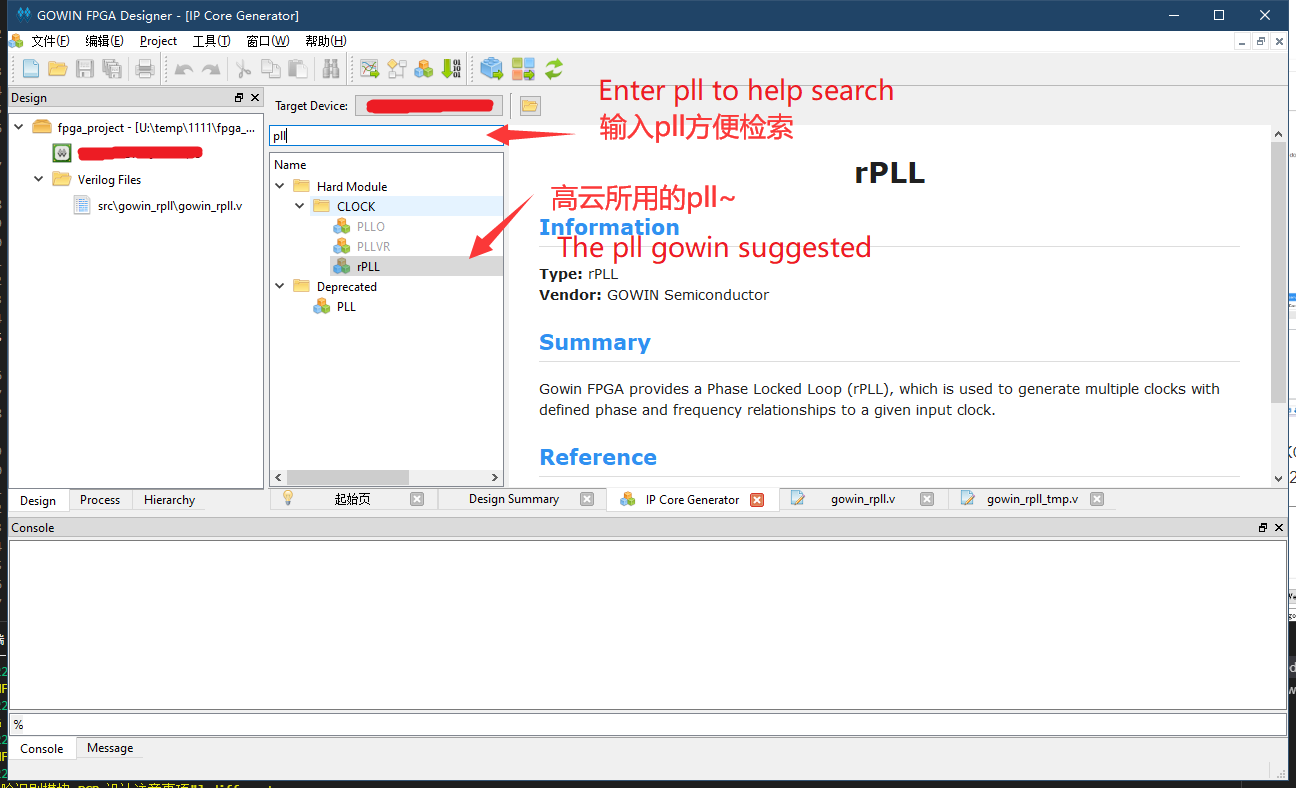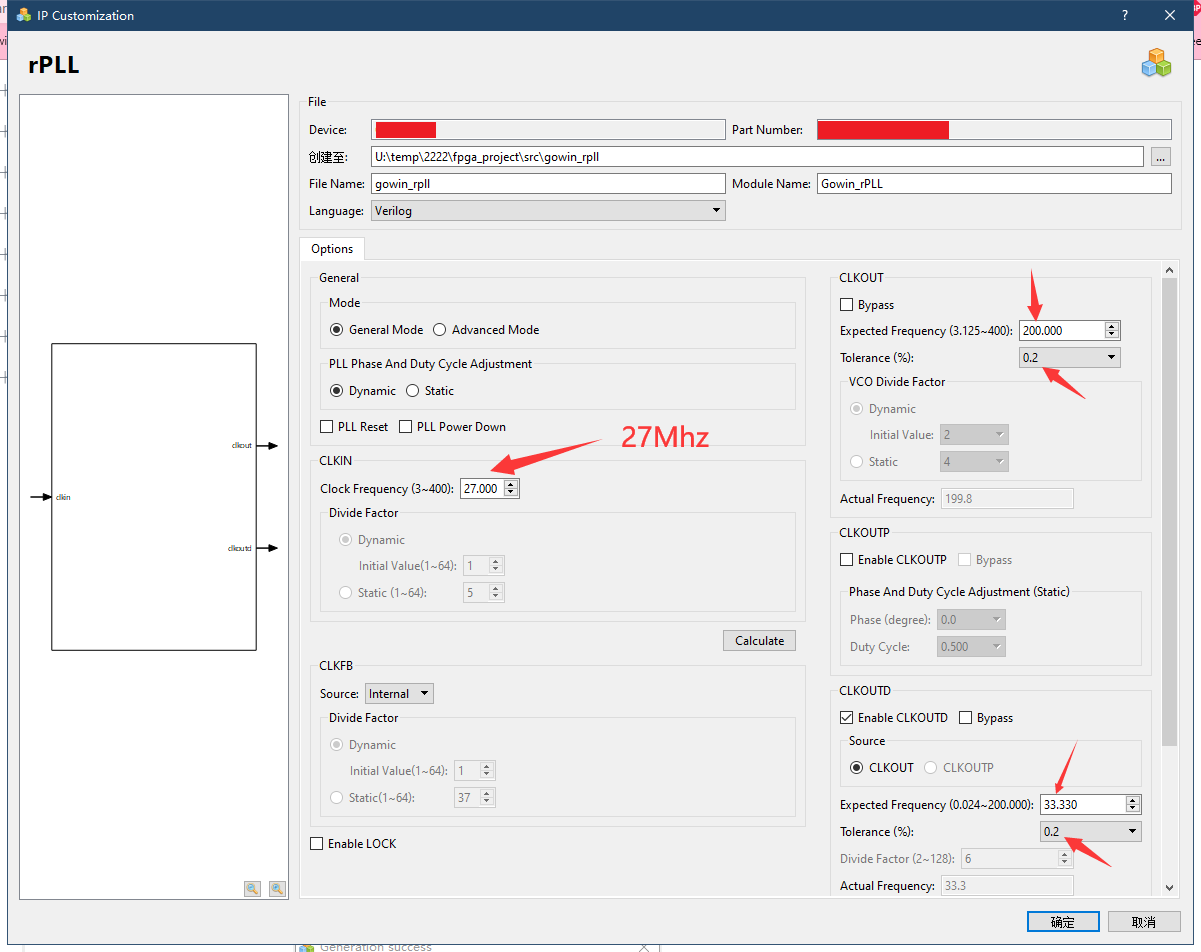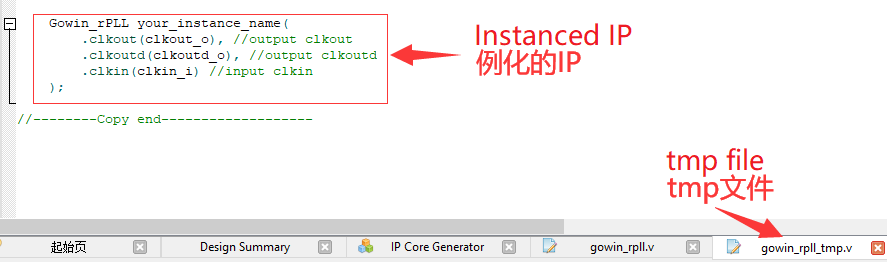## 屏幕驱动代码

• 首先新建一个额外的verilog文件来保存下面要编写的代码

### 端口定义

module VGAMod
(
input                   CLK,
input                   nRST,

input                   PixelClk,

output                  LCD_DE,
output                  LCD_HSYNC,
output                  LCD_VSYNC,

output          [4:0]   LCD_B,
output          [5:0]   LCD_G,
output          [4:0]   LCD_R
);

### 时序常量

localparam      V_BackPorch = 16'd6; //0 or 45
localparam      V_Pluse     = 16'd5;
localparam      HightPixel  = 16'd480;
localparam      V_FrontPorch= 16'd62; //45 or 0

localparam      H_BackPorch = 16'd182;
localparam      H_Pluse     = 16'd1;
localparam      WidthPixel  = 16'd800;
localparam      H_FrontPorch= 16'd210;

localparam      PixelForHS  =   WidthPixel + H_BackPorch + H_FrontPorch;
localparam      LineForVS   =   HightPixel + V_BackPorch + V_FrontPorch;

### 计数变量

• 定义一些变量能够容易编写程序

reg [15:0] LineCount;
reg [15:0] PixelCount;

reg [9:0]  Data_R;
reg [9:0]  Data_G;
reg [9:0]  Data_B;

### 同步信号

    always @(  posedge PixelClk or negedge nRST  )begin
if( !nRST ) begin
LineCount       <=  16'b0;
PixelCount      <=  16'b0;
end
else if(  PixelCount  ==  PixelForHS ) begin
PixelCount      <=  16'b0;
LineCount       <=  LineCount + 1'b1;
end
else if(  LineCount  == LineForVS  ) begin
LineCount       <=  16'b0;
PixelCount      <=  16'b0;
end
else
PixelCount      <=  PixelCount + 1'b1;
end

always @(  posedge PixelClk or negedge nRST  )begin
if( !nRST ) begin
Data_R <= 9'b0;
Data_G <= 9'b0;
Data_B <= 9'b0;
end
else begin
end
end

//注意这里HSYNC和VSYNC负极性
assign  LCD_HSYNC = (( PixelCount >= H_Pluse)&&( PixelCount <= (PixelForHS-H_FrontPorch))) ? 1'b0 : 1'b1;
assign  LCD_VSYNC = ((( LineCount  >= V_Pluse )&&( LineCount  <= (LineForVS-0) )) ) ? 1'b0 : 1'b1;

### 使能信号

assign  LCD_DE = (  ( PixelCount >= H_BackPorch )&&
( PixelCount <= PixelForHS-H_FrontPorch ) &&
( LineCount >= V_BackPorch ) &&
( LineCount <= LineForVS-V_FrontPorch-1 ))  ? 1'b1 : 1'b0;
//这里不减一，会抖动

### 测试彩条

assign  LCD_R   =   (PixelCount<200)? 5'b00000 :
(PixelCount<240 ? 5'b00001 :
(PixelCount<280 ? 5'b00010 :
(PixelCount<320 ? 5'b00100 :
(PixelCount<360 ? 5'b01000 :
(PixelCount<400 ? 5'b10000 :  5'b00000 )))));

assign  LCD_G   =   (PixelCount<400)? 6'b000000 :
(PixelCount<440 ? 6'b000001 :
(PixelCount<480 ? 6'b000010 :
(PixelCount<520 ? 6'b000100 :
(PixelCount<560 ? 6'b001000 :
(PixelCount<600 ? 6'b010000 :
(PixelCount<640 ? 6'b100000 : 6'b000000 ))))));

assign  LCD_B   =   (PixelCount<640)? 5'b00000 :
(PixelCount<680 ? 5'b00001 :
(PixelCount<720 ? 5'b00010 :
(PixelCount<760 ? 5'b00100 :
(PixelCount<800 ? 5'b01000 :
(PixelCount<840 ? 5'b10000 :  5'b00000 )))));

## 在顶层模块中例化

• 这里也是要新建文件的
module TOP //设置顶层模块
(
input           nRST,
input           XTAL_IN,

output          LCD_CLK,
output          LCD_HYNC,
output          LCD_SYNC,
output          LCD_DEN,
output  [4:0]   LCD_R,
output  [5:0]   LCD_G,
output  [4:0]   LCD_B

); // 罗列需要的端口

wire        CLK_SYS;
wire        CLK_PIX;

//例化pll
Gowin_rPLL chip_pll(
.clkout(CLK_SYS), //output clkout     //200M
.clkoutd(CLK_PIX), //output clkoutd   //33.00M
.clkin(XTAL_IN)    //input clkin
);

VGAMod  VGAMod_inst //例化vga驱动
(
.CLK        (   CLK_SYS     ),
.nRST       (   nRST        ),

.PixelClk   (   CLK_PIX     ),
.LCD_DE     (   LCD_DEN     ),
.LCD_HSYNC  (   LCD_HYNC    ),
.LCD_VSYNC  (   LCD_SYNC    ),

.LCD_B      (   LCD_B       ),
.LCD_G      (   LCD_G       ),
.LCD_R      (   LCD_R       )
);

assign      LCD_CLK     =   CLK_PIX;

endmodule

## 综合、约束、布局布线

### 综合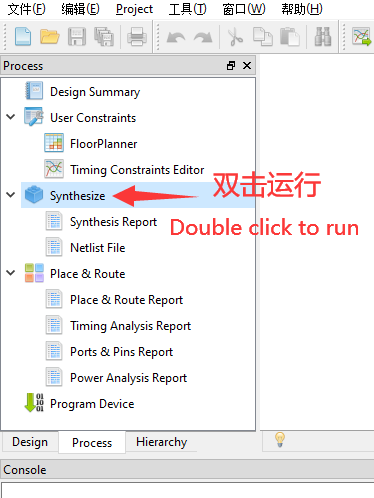### 约束

• 此处仅管脚约束

PORT PIN PORT PIN
LED_B 10 LED_G 11
LED_R 9 LCD_B 24
LCD_B 23 LCD_B 22
LCD_B 20 LCD_B 19
LCD_G 18 LCD_G 17
LCD_G 16 LCD_G 15
LCD_G 27 LCD_G 28
LCD_R 29 LCD_R 30
LCD_R 31 LCD_R 34
LCD_R 35 LCD_DEN 21
LCD_SYNC 32 LCD_HYNC 33
LCD_CLK 8 XTAL_IN 47
nRST 44

### 布局布线